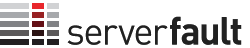## Votes per post broken down

0

(click on this box to dismiss)Q&A for system and network administrators

``````SELECT
COUNT(distinct p.id) as posts,
CAST(
CAST(COUNT(CASE when v.VoteTypeId = '2' then v.id end) as DECIMAL(20,5))
/
CAST(COUNT(distinct p.id) as DECIMAL(20,5))
as DECIMAL(20,5)) as voteweightUp,
CAST(
CAST(COUNT(CASE when v.VoteTypeId = '3' then v.id end) as DECIMAL(20,5))
/
CAST(COUNT(distinct p.id) as DECIMAL(20,5))
as DECIMAL(20,5)) as voteweightDown
FROM posts pHold tight while we fetch your results